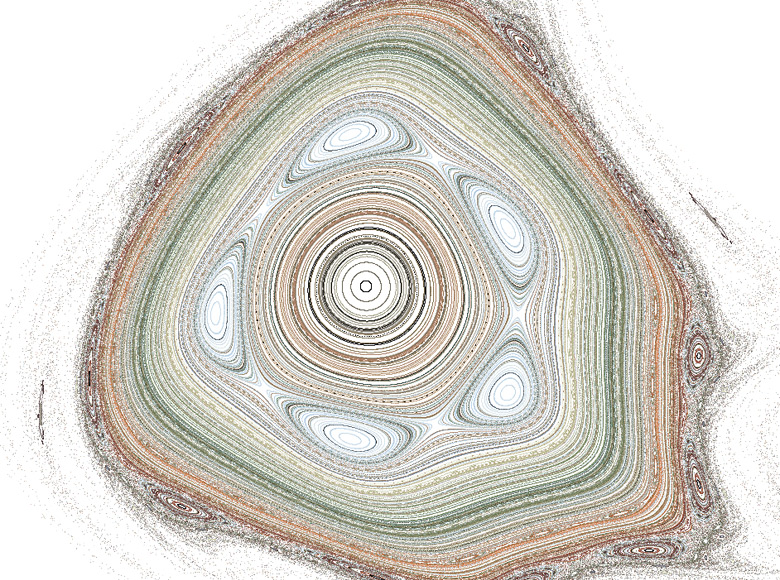processing    march, 2004    j.tarbell
based on code by Paul Bourke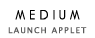200 x 200 pixelsIn computing time scales, the Henon Phase is an ancient strange attractor. A point is moved through two dimensional space based on the following transformational equations: xn+1 = xn cos(a) - (yn - xn2) sin(a) yn+1 = xn sin(a) + (yn - xn2) cos(a) These images plot the phase space of the Henon system at arbitrary values of a.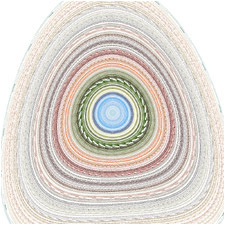00000001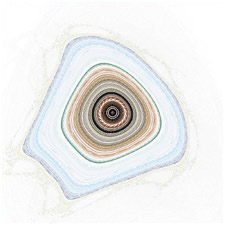0002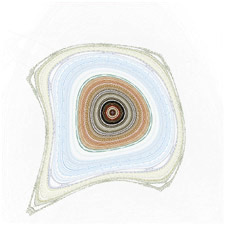0003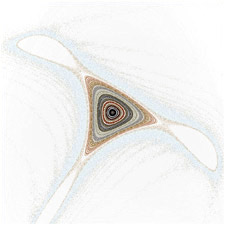0004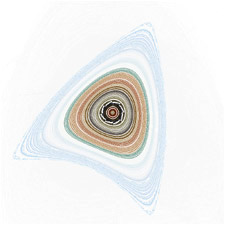0005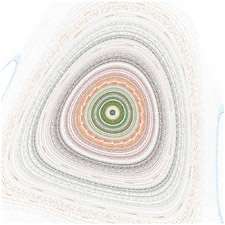0006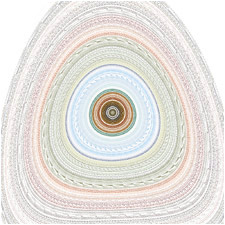000700080009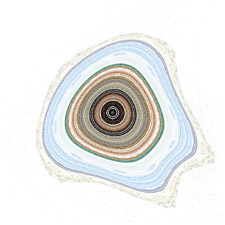0010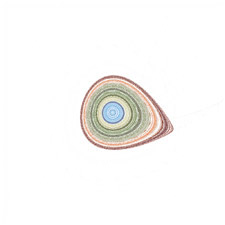0011